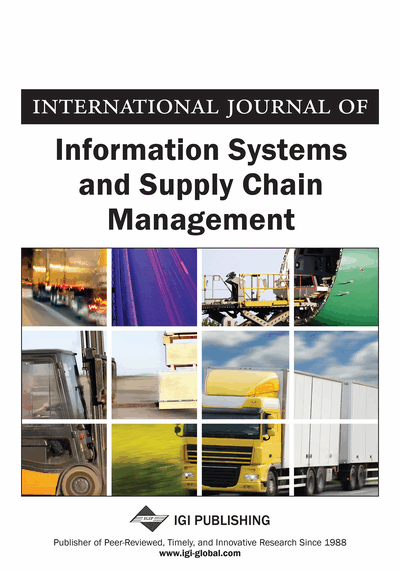# Supplier Selection Under Conditions of Uncertainty

Farouq Alhourani (University of Tampa, Tampa, USA) and Umesh Saxena (University of Wisconsin-Milwaukee, Milwaukee, USA)
DOI: 10.4018/IJISSCM.2019100103
Available
\$37.50
No Current Special Offers

## Abstract

Supplier selection is one of the critical decision-making problems in supply chain management. The purpose of this research is to investigate the process that is commonly used for calculating a “score” for each supplier when certain attributes are used. In decision making theory, the selection of “best” alternative can be done under three different conditions: certainty, risk, and uncertainty. Most of the current research uses “certainty” conditions. This article suggests that the “uncertainty” condition should be used in supplier selection as it corresponds to real-life more than the certainty or risk condition. “Maximum entropy” method is used to ascertain the probability function of the outcomes. Once the probability functions are known, means and variance can be calculated, and the suppliers can be evaluated.
Article Preview
Top

## 2. Research Objective

The purpose of this research is to investigate the process that is commonly used for calculation of a “score” for each supplier using a known number of attributes. The score is then used for selection of suppliers. Many investigators have used the following linear model to calculate this score.

Sj = ∑ Wi Xij(1)

where Sj is the score for the jth supplier

Wi is the weight or relative importance of ith attribute

Xij is the value of ith attribute for jth supplier

n is the number of attributes

Use of equation 1 requires that the values of both Wi and Xij are known. Attention is mainly focused on the process by which the values of Xij may be obtained. It is worth mentioning that it is very difficult to estimate these values precisely as these are routinely measured on an ordinal scale.

Classical decision-making problems deal with the selection of “best” alternative from a list of alternatives. Selection of a supplier is clearly a type of decision-making problem. In decision making theory, the selection of “best” alternative can be done under three different conditions: certainty, risk, and uncertainty. Certainty assumes that an outcome can take only one value. Underlying assumption under conditions of risk is that probability function of outcomes follows a known probability function. As an example, if probability function is “normal” then its mean and variance are known or can be estimated. In uncertainty, the decision maker knows the outcomes, but probability of each outcome is not known.

## Complete Article List

Search this Journal:
Reset
Open Access Articles
Volume 15: 4 Issues (2022): 1 Released, 3 Forthcoming
Volume 14: 4 Issues (2021): 2 Released, 2 Forthcoming
Volume 13: 4 Issues (2020)
Volume 12: 4 Issues (2019)
Volume 11: 4 Issues (2018)
Volume 10: 4 Issues (2017)
Volume 9: 4 Issues (2016)
Volume 8: 4 Issues (2015)
Volume 7: 4 Issues (2014)
Volume 6: 4 Issues (2013)
Volume 5: 4 Issues (2012)
Volume 4: 4 Issues (2011)
Volume 3: 4 Issues (2010)
Volume 2: 4 Issues (2009)
Volume 1: 4 Issues (2008)
View Complete Journal Contents Listing